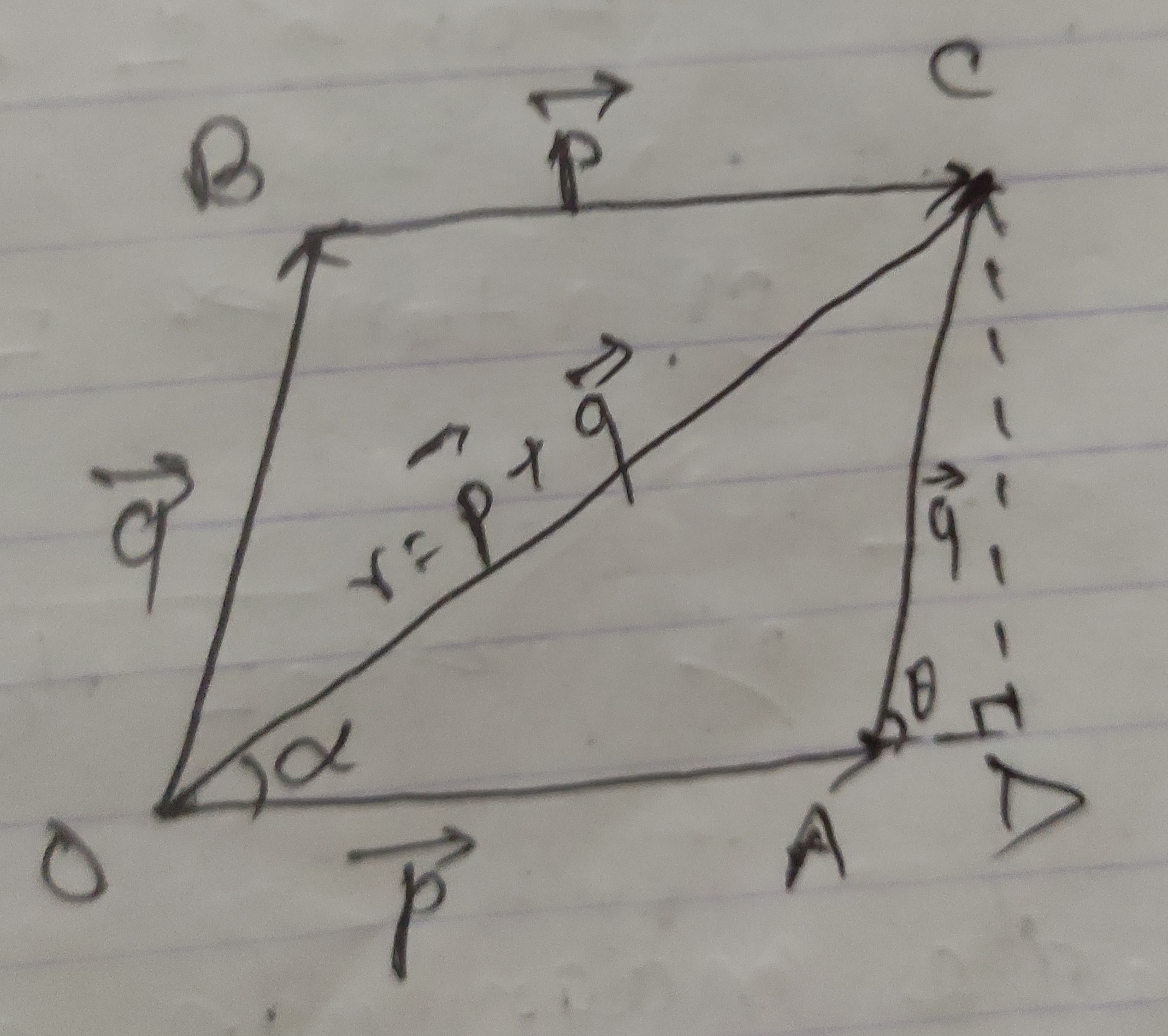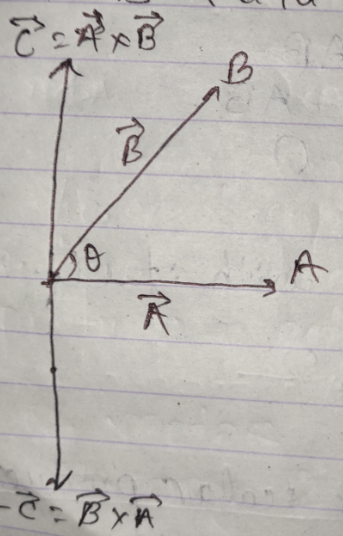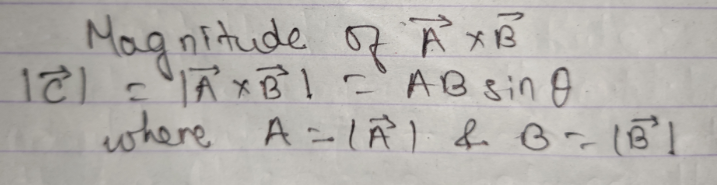VectorsVector: The physical quantities which require both magnitude and direction for complete description are called vectors. eg:- moment, impulse, angular displacement, angular velocity, angular acceleration, torque, magnetic moment, gradient of any quantity, length element, area element, etc.

Scalar: The physical quantities which require only magnitude for complete description are called scalar quantity. eg:- length, distance, area, flux of vector quantity, intensity of radiation, electrical charge, electrical current, electrical potential, etc.

Tensors: Physical quantities having no fixed direction but different magnitude at different points are called tensors. eg:- moment of inertia, electrical conductivity, dielectric constant, refractive index, etc.

Condition for a quantity to be a vector:

Necessary Condition

The quantity should have a particular direction.

Sufficient Condition

Types of vector:

1. Polar(Real) vectors: Vectors starting from a point or having start point(as in case of displacement) or point of application(as in case of force) is called polar(real) vector. They have natural direction and unidirectional motion. eg:- displacement, velocity, acceleration, momentum, impulse, force, etc.
2. Axial(Pseudo) vectors: Vectors representing rotation and are along the axis of rotation in accordance with right hand's screw or thumb rule is called axial(pseudo) vectors.They have no natural direction. Its direction is along the direction of axis of rotation. eg:- angular displacement, angular velocity, angular acceleration, torque, magnetic moment, etc.
3. Zero(Null) vector: A vector that has 0 magnitude is called zero or null vector.
4. Unit vectors: A unit vector of a given number is a dimensionless vector that has a magnitude of 1 and has same direction as of the given vector. Since, vectors are infinite in number , unit vectors are also infinite in number. It is denoted by Â(read as A cap).
5. Displacement vectors: The vector from the initial point to the final point is called displacement vector.
6. Co-initial vectors: The vectors having same initial pointare called co-initial vectors.
7. Co-terminus vectors: The vectors having same final or terminalpoint are called co-terminus vectors.
8. Position vectors: The vector from the origin to the point on co-ordinate is called position vector.
9. Parallel vectors: Two vectors A and B are said to beparallel vectors if their directions are parallel or they are in same direction.
10. Negative vectors: The negative of a vector A is the vectorwhose magnitude is equal but direction is opposite to that of A. It is denoted by -A vector.
11. Equal vectors: Two vectors A and B are said to be equal if they have the same magnitude and the same direction.
12. Collinear vectors: Two vectors A and B are said to becollinear if they lie on they pass through the same line.
13. Co-planar vectors: Vectors that lie on the same plane are called co-planar vectors.
14. Like vector,
15. Unlike vector,
16. Proper vector, etc.

Composition of vectors: The process of combining two or more vectors into a single vector which is the resultant of them is called composition of vectors.

Resultant of vectors or resultant vectors: A single vector that produces same effect as is produced by individual vectors together is called resultant of vectors or resultant vector.

Angle between two vectors: The angle between two vectors is measured by putting their tails together. If 2 vectors are not acting on a point, then angle between them can be obtained by displacing one of the vector parallel to itself so that the tails of 2 vector meets at a point.Here, the angle between vectors A and B is the the angle β.

Vector Representation:

One way to represent a vector is by means of an arrow. The direction of the arrow is the direction of the vector and the length of the arrow, drawn to a scale represents its magnitude.If 2 vectors, P and Q are represented in magnitude and direction by 2 adjacent sides of a triangle taken in same order then their resultant vector, r(= p+q) is represented in both magnitude and direction by the 3rd side of the triangle in opposite order.

Let OA and AB are two p and q vectors respectively.The resultant vector, r(= p+q) makes an angle α with the vector p. We produce OA and OC and draw BC perpendicular to OC. Let the q makes an angle Ɵ with OC.The magnitude and direction of the resultant vector (r) of vectors p and q is  andwith respect to vector p respectively.

If 2 vectors acting simultaneously at a point can be represented by 2 adjacent sides of a parallelogram drawn from a point then their resultant is represented in both magnitude and direction by the diagonal of parallelogram drawn from that point.

Let 2 vectors OA(p) and OB(q) initializing from the samepoint O acting in such a way that these 2 vectors represent the 2 adjacent sides of a parallelogram, then the resultant of these 2 vectors, r(= p+q), vector OC is represented by the diagonal of the parallelogram from the same initial point. Vector OA is produced to OD such that CD is drawn perpendicular to OD. Resultant vector r and vector q makes angle α andwith OD respectively.The magnitude and direction of the resultant vector (r) of vectors p and q isandwith respect to vector p respectively.

If a number of represented in both magnitude and direction by (n - 1) sides of n sided polygon taken in same order, then the nth side (closing side) of this polygon taken in opposite order represents the resultant of them in both magnitude and direction.Law of Algebra for the Vector Sum:

Commutative Law:

i.e.,Associative Law:

i.e.,Subtraction of Vectors

The process of adding of a vector with negative of another vector is called vector subtraction. In order to find out the magnitude and direction of vector a-b, we reverse the direction of vector b thus producing –b vector which is added to vector a and the resultant vector is the subtraction of vector b from a.Multiplication of Vectors

Multiplication of a Vector by a Scalar

If vector A is multiplied by a scalar quantity m, the product mA is a vector that has same direction as A and magnitude m×|A|.

The direction may change as follows:

1. The direction of the product mA is the same as A if m is positive.
2. The direction of the product mA is undefined if m is zero(0).
3. The direction of the product mA is the same as -A if m is negative.

Laws of Algebra for Scalar Multiple

1. Associative law:                                                                                                                                            i.e.,2. Distributive law:                                                                                                                                  i.e.,Multiplication of a Vector by a Vector

There is 2 kind of multiplication of a vector by a vector:

• Scalar or dot product:

Multiplication of a vector by a second vector by a second vector so as to produce a scalar is called scalar or dot product of given 2 vectors.

Consider vectors A and B with an angle Ɵ betweenthem as shown in figure. Then, the scalar product of them is given bySince, A, B, cosƟ are scalars, product of vectors A and B is also scalar. Being scalar it has no direction.

It is also defined as the product of magnitude of one vector and scalar components of second vector along the direction of first vector.Properties of Dot Product:Laws of Algebra for Dot Product:• Cross or vector product:

Multiplication of a vector by another vector so as to produce another vector is called cross product of 2 vectors.

It is denoted by cross(×) between 2 vectors. It has both magnitude and direction, so it is also called vector product.

Consider vectors A and B with smaller angle Ɵbetween them. Then the cross product of vectors A and B is given by vector C(= A×B). It is a vector because C is itself a vector. So, it has both magnitude and direction.Direction of vector A×B

Its direction is along the line perpendicular to the plane containing vector A and vector B and is given by right hand thumb rule.

Properties of Cross ProductLaws of Algebra for Cross Product:Division of Vectors

The division of any 2 vectors is not possible.

Right Hand's Thumb Rule:

Curl the fingers of the right hand so that it would rotate first vector into the second vector through the smaller angle between them then the extended thumb gives the direction of vector C(= A×B).Where n is unit normal vector along the direction of vector A×B which is perpendicular to both the vectors and to the plain containing two vectors.Resolution of Vectors: The process of splitting a vector into 2 or more vectors in different directions on a plane such that their sum gives back the original vector is called the resolution of vectors.

The splitted vectors are called components of the vector.